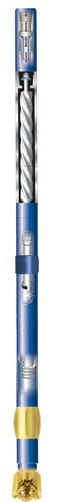# Total Bit Revolution in When Running A Drilling Mud Motor and Rotating At Surface

I’ve got an email asking me about how to figure out the total bit revolution (RPM) at down hole when the mud motor is used in the drill string  and the rotating at surface is also conducted.There are two components contributing in the total revolution of downhole drilling bit using mud motor.

1. The rotor RPM of drilling mud motor– each drilling mud motor has its specific performance data which will tell you how many revolutions per flow rate flowing through it.

For example (see the image below), it shows Rev/Gal which means the rotor will turn 0.147 turn/min per one gallon/min of drilling mud passing thought.2. The rotary speed from top drive or rotary table.

The total drilling bit revolution downhole:

The total drilling bit revolution is equal to summation of the rotor RPM at specific flow rate and the rotary speed on surface.

Please follow the example below to determine the bit revolution down hole.

The performance data of mud motor –

Rev/Gal = 0.147

Flow rate = 320 gpm

Rotary speed = 220 rpm

The rotor rpm based on 320 gpm = 0.147 x 320 = 47 rpm

Total bit revolution down hole = 47 + 220 = 267 revolution per minute (rpm)

Share the joyWorking in the oil field and loving to share knowledge.

### 4 Responses to Total Bit Revolution in When Running A Drilling Mud Motor and Rotating At Surface

1.Azer says:

Bit revolution depends on Mud Motor Type, Flow Rate, Rotary RPM and Hrs.
in order to calculate together with hours I am using this formula:

( Flow Rate x Rev/Gal x 60 x ( Oriented drilling hrs + Circulating hrs ) +
( Flow Rate x Rev/Gal + Rotary RPM ) x 60 x ( Rotary Drilling hrs + Reaming hrs )

•DrillingFormulas.Com says:

The formula that you show me is the total bit rotation.

2.Mika Myers says:

Any idea how to calculate the “Real Efficiency” of a mud motor as differential increases?
I am interested in knowing this formula.

Note: Read the rpm from a motor performance curve supplied by the manufacturer. RPM decreases exponentially as motor differential increases.

3.Manmohan Sharma says:

The page was informative & useful. The formula was made quite clear.

This site uses Akismet to reduce spam. Learn how your comment data is processed.